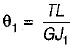Courses

# Test: Torsion of Shafts - 3

## 10 Questions MCQ Test Topicwise Question Bank for Mechanical Engineering | Test: Torsion of Shafts - 3

Description
This mock test of Test: Torsion of Shafts - 3 for Mechanical Engineering helps you for every Mechanical Engineering entrance exam. This contains 10 Multiple Choice Questions for Mechanical Engineering Test: Torsion of Shafts - 3 (mcq) to study with solutions a complete question bank. The solved questions answers in this Test: Torsion of Shafts - 3 quiz give you a good mix of easy questions and tough questions. Mechanical Engineering students definitely take this Test: Torsion of Shafts - 3 exercise for a better result in the exam. You can find other Test: Torsion of Shafts - 3 extra questions, long questions & short questions for Mechanical Engineering on EduRev as well by searching above.
QUESTION: 1

### For a circular shaft of diameter d subjected to torque T, the maximum value of the shear stress is

Solution:

From the equation of torsion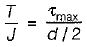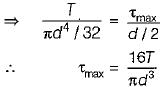QUESTION: 2

### A stepped steel shaft shown below is subjected to 10 N-m torque. If the modulus of rigidity is 80 GPa, the strain energy in the shaft in N-mm is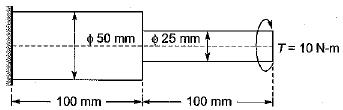Solution:

The strain energy in the shaft is 1.73 N mm.

Given-

• Torque = 10 Nm
• Modulus of rigidity = 80 GPa

From the figure we can say that

T₁ = T₂ = T = 10 Nm

Here

J₁ = π/32 d⁴

By substituting the value we get

J₁ = (π/32) × 25⁴ × 10⁻¹²

G₁ = 80 × 10⁹

l = 0.1 m

θ₁ = 0.33 × 10⁻³

So,

T₁ = J₁G₁θ₁/l₁ ⇒ θ₁ = T₁l₁/J₁G₁

Hence,

θ₁/θ₂ = (J₂/J₁) = l₁/l₂ = G₂/G₁

Now according to the question

θ₁ = 4 θ₂ So,

θ = θ₁ + θ₂ = 5/4 × 0.33 × 10⁻³

Now strain energy can be given by

Strain energy = 1/2 Tθ

By substituting the value we get

Strain energy = 1/2 × 10 × 5/4 × 0.33 × 10⁻³ = 1.73

QUESTION: 3

### While transmitting the same power by a shaft, if its speed is doubled, what should be its new diameter if the maximum shear stress induced in the shaft remains same?

Solution: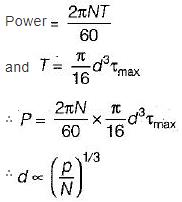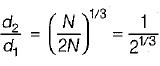QUESTION: 4

A solid shaft of diameter 100 mm, length 1000 mm is subjected to a twisting moment ‘T’, the maximum shear stress developed in the shaft is 60 N/mm2. A hole of 50 mm diameter is now drilled throughout the length of the shaft. To developed a maximum shear stress of 60 N/ mm2 in the hollow shaft, the torque ‘ T must be reduced by

Solution:

For solid shaft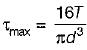............... (i)
for hollow shaft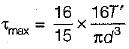.................. (ii)
From (i) and (ii), we get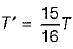Hence reduced torque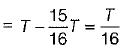QUESTION: 5

Maximum shear stress developed on the surface - of a solid circular shaft under pure torsion is 240 MPa. If the shaft diameter is doubled then the maximum shear stress developed corresponding to the same torque will be

Solution: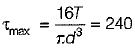When shaft diameter is doubled then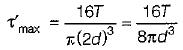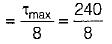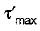= 30 MPa

QUESTION: 6

A circular shaft is subjected to a twisting moment T and bending moment M. The ratio of maximum bending stress to maximum shear stress is given by

Solution:

Maximum bending stress,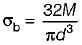Maximum shear stress,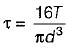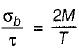QUESTION: 7

A section of a solid circular shaft with diameter D is subjected to bending moment M and torque T. The expression for maximum principal stress at the section is

Solution:

Principal stresses,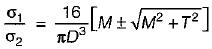Maximum principal stress is obtained by choosing '+' sign and minimum principal stress is be obtained by '-' sign.

QUESTION: 8

Two shafts, one of solid section and the other of hollow section, of same material and weight having same length are subjected to equal torsional force. What is the torsional stiffness of hollow shaft?

Solution:

Let the diameter of solid shaft be ds. Let the external and internal diameter of the hollow shaft be d0 and di respectively.
It is given that both the shafts are made of same material, have same weight and length and are subjected to equal torsional force.
∴ Weight of solid shaft = Weight of hollow shaft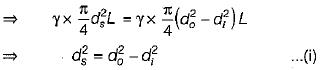Now, we know that, torsional stiffness is given as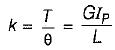For same value of G and L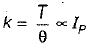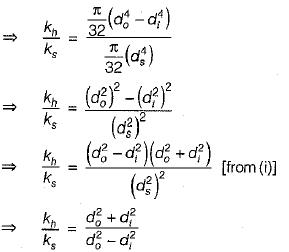Now, the quantity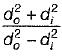is always greater than 1
∴ K> Ks

QUESTION: 9

A long shaft of diameter d is subjected to twisting moment T at its ends. The maximum normal stress acting at its cross-section is equal to

Solution:

Maximum shear stress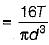Normal stress = 0

QUESTION: 10

A circular shaft shown in the figure is subjected to torsion T at two points A and B. The torsional rigidity of portions CA and BD is GJ1 and that of portion AB is GJ2. The rotations of shaft at points A and B are q1 and q2. The rotation q1 isSolution:

The symmetry of the shaft shows that there is no torsion on section AB.
∴ Rotation,